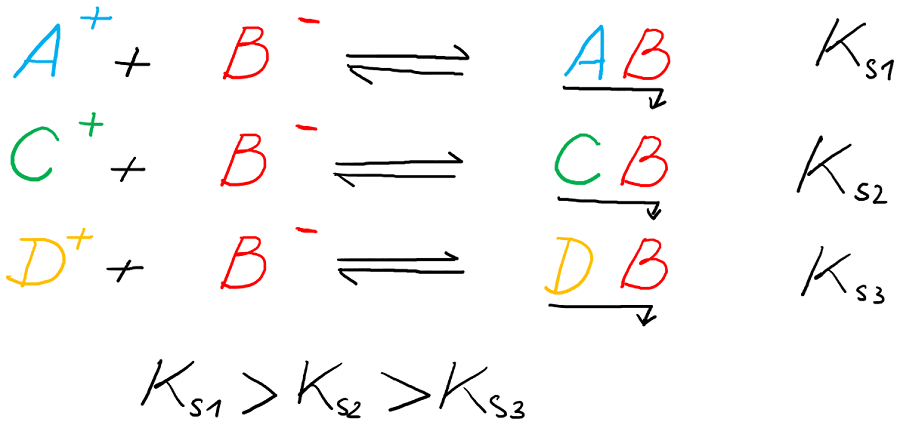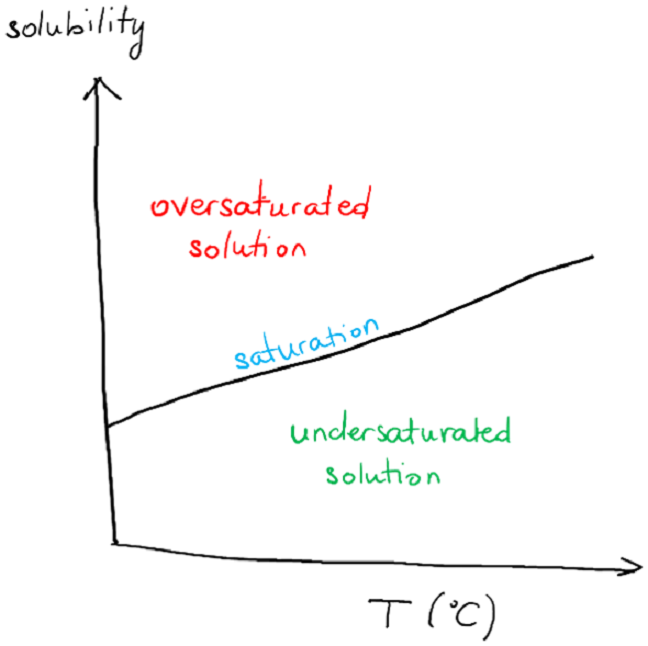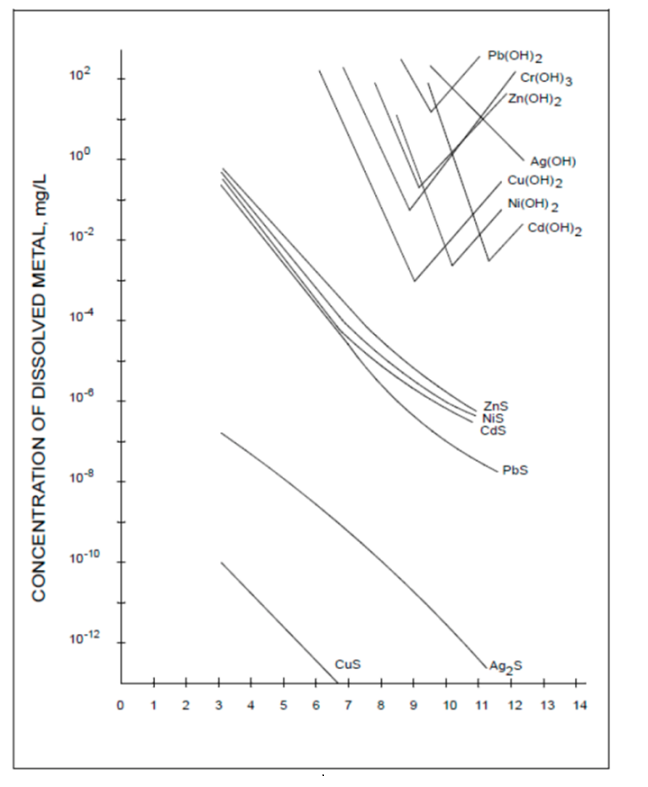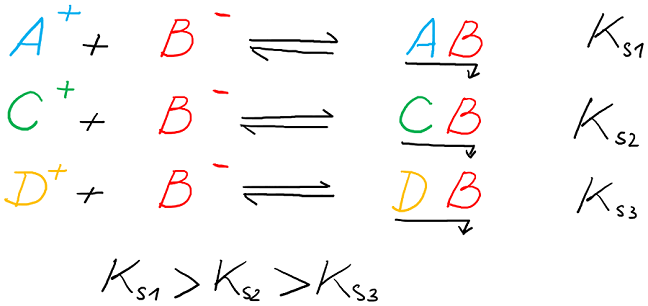New offer! Get 30% off your first 2 months of Unlimited Monthly. Start your subscription for just £29.99 £19.99. New subscribers only. T&Cs apply

•# Different types of metal precipitation

What are the principles of metallic precipitation? Under what conditions can precipitation occur? Read Domitille Giaume's explanation.This article presents the principle of metallic precipitation and conditions under which precipitation can occur: oversaturation, addition of reagents to form an insoluble salt, addition of reagents to form an insoluble metallic compound: cementation. Finally, the principle of fractional separation is presented.

Precipitation is a separating technique that can be used in extraction and recycling. This can be done either

• to perform selective removal of metallic impurities by precipitating them or
• to purify a metal by selectively precipitating it out of a solution containing multiple elements

Precipitation can be performed by physical techniques as well as by chemical ones. For the chemical method, precipitation can have different origins.

## 1. Principle of precipitation

The reason for a compound to precipitate is its solubility limit reached in the solvent, here water. The solubility of a compound can be defined as the maximum weight of this compound that can be dissolved in 1 litre of a solvent (most of the case, water). Depending on the temperature, the solubility might change, as shown on Figure 1.Fig. 1: Solubility as a function of temperature

On Figure 1, below the blue line corresponding to the solubility of the compound AB, the solution is unsaturated: all AB compound can be dissolved in solution; above the blue line, the solution is oversaturated: AB has been dissolved to reach a saturated solution but solid AB is still present, as no more solid can be dissolved in the solution over the saturation.

The solubility reaction of a compound AB can be written as follows:

[A_aB_b (s) leftrightarrow aA^{b+}(l)+bB^{a-} (l)]

with the solubility product

[K_S=[A^{b+}]^{a}[B^{a-}]^{b}]

This reaction is an equilibrium: from the solid to dissolved ions (from left to right), this is a dissolution; and from the ions to the solid (from right to left), this is precipitation. This equilibrium is characterized by a solubility product constant Ks. The higher the solubility product constant, the more soluble the solid.

Thus, starting from a solution containing the two ions Ab+ and Ba- at the concentrations [A0]and [B0], it is possible to determine the appearance of a precipitate by comparing

the ionic product (Q=[A_0^{b+}]^{a}[B_0^{a-}]^{b})

with the equilibrium (K_S).

• As long as (Q < K_S), no precipitate is observed.
• If (Q > K_S), this means that the initial solution is oversaturated, and solid (A_aB_b) will precipitate, in equilibrium with a saturated solution. This precipitated (A_aB_b) solid can be separated by filtering but leaves in the solution the ions at the concentration of equilibrium.

## 2. Conditions to reach precipitation

There are different ways to reach this condition of precipitation Q> Ks.

### 2.1. Solvent evaporation

The first possibility to reach the conditions of precipitation is to simply evaporate the solvent, thus increasing all the concentrations of the elements present in solution. The condition of oversaturation is reached at one point, leading to the precipitation of salts. This is typically the principle of NaCl extraction from seawater, or lithium chloride extraction out of Salars .

### 2.2. Addition of reagents to form an insoluble salt.

Instead of increasing the concentrations of all the elements present in solution, another possibility is to add a reagent in the solution that will react with a specific metallic element in the solution, forming an insoluble salt. This salt can then be removed out of the solution.

As an example, Nickel-rich leachates obtained from Ni-Mo ores contain multiple impurities, among which copper. A very efficient solution to purify this leachate from copper impurities is to precipitate copper as its sulfide. This is possible by the addition in solution of nickel sulphide NiS, which can be solubilized due to high constant product constant. CuS present a lower Ks as presented in Figure 1 . The following precipitation reaction can then occur, after NiS dissolution:

Cu2+ (aq) + S2- (aq) → CuS((sl)Fig. 2: Precipitation pH as a function of concentration of hydroxides and sulfides.

### 2.3. Addition of reagents to form an insoluble metallic compound: cementation

Cementation is a specific term used in mineral extraction process or in recycling industry to name the redox reaction between a solubilised metallic ion and a solid metal. It consists in a redox reaction, during which a metallic salt of the solution is reduced into its metallic entity. At the same time, the metallic powder is solubilized in the solution. The driving force of cementation is the redox potential difference between one cationic entity of the solution and the solid metal added, leading to the reduction of the metallic ion into its metal entity, and the oxidation of the solid metal into its solubilized cation.

This method is widely used during hydrometallurgy extraction from ores, as after the lixiviation process, the mineral or the wastes are dissolved all together in an acidic solution. Cementation can allow the recovery of a pure precipitate, or purify a solution from impurities.

The metal obtained by cementation can be filtered and recovered, which is typically the case during copper extraction from sulphide ores. The copper salts are first extracted by oxidative leaching from enriched ores. This produces a dilute solution of copper sulphate. Cementation is then done by injecting the copper solution through an iron bed, usually waste material such as cans. The copper precipitates by the redox reaction:

Cu2+(aq) + Fe(s) → Cu(s) + Fe2+(aq)

This process allow the recovery of pure metallic copper.

As another application, the metallic impurities present in a solution can be purified out of this solution by cementation. In the case of zinc extraction from zinc sulphide ores, a solution of ZnSO4 is obtained after lixiviation and hydrolysis process. The solution also contains multiple impurities such as Co2+, Cu2+, Ni2+ metallic ions, initially present in the ores. A simple addition in the solution of Zinc metallic powder allows their elimination out of the solution by redox reaction:

Mz+(aq) + z/2 Zn(s) → M(s) + z/2 Znz+(aq)

## 3. Fractional separation

When multiple cations are present in solution, competitive reactions might occur with a same anion, leading to different precipitates. This anion can be either already present in solution, or added as another salt. By a sharp control of this anion concentration, fractional separation of the cations can be performed. Assuming that 3 cations can form precipitates with the same anion, and are present together in the same solution, a fractional precipitation can be performed by controlling the anion concentration.Let us assume that a solution contains cations A+, C+ and D+ at the same initial concentrations of [A0], [C0] and [D0], with [A0]=[C0]=[D0]. Let us also take a case where Ks1 > Ks2 > Ks3.

To precipitate the AB solid, the concentration of B should be above Ks1/[A0]. Similarly, to precipitate CB, the concentration of B should be above Ks2/[C0]; and to precipitate DB, the concentration of B should be above Ks3/[D0].

Then upon addition of B in the solution, DB will precipitate first, then CB and finally AB. The control of B concentration in the solution and multiple steps of filtering allow fractional separation of the different cations by precipitation. The purity of the precipitates will depend on the difference between the Ks values.